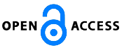Isaac Scientific Publishing### Common Coupled Fixed Points for Four Maps Using Property(E.A.) in Complex Valued b- Metric Spaces

Download PDF (523.5 KB) PP. 1 - 7 Pub. Date: July 8, 2016

### Author(s)

• K.P.R.Rao*
Department of Mathematics, Acharya Nagarjuna University, Nagarjuna Nagar -522 510, A.P., India.
Department of Mathematics, Sir C R R College of Engineering, Eluru, West Godhawari - 534007, Andhra Pradesh, India
• S.V.K.Sarma
Department of S and H(Mathematics), Dhanekula Institute of Engineering and Technology, Ganguru, Penamaluru Mandalam, Vijayawada -521139

### Abstract

In this paper, we obtain a unique common coupled fixed point theorem for four self maps using property(E.A.)in complex valued b-metric spaces. Also we give an example to illustrate our main theorem.

### Keywords

Complete metric spaces, property(E.A), w-compatible mappings

### References

 A.Azam,B.Fisher and M.Khan,Common fixed point theorems in complex valued metric spaces, Numer. Funct. Anal. Optim. 32(3), (2011), 243-253.

 C.Klin-eam and C.Suanoom,Some common fixed point theorems for generralized contractive type mappings on complex valued metric spaces, Abstr.Appl.Anal.,2013,6 pages,Article ID 604215, doi.10.1155/2013/604215.

 F. Rouzkard and M. Imdad, Some common fixed point theorems on complex valued metric spaces, Comp. Math.Appls., 64,(2012), 1866-1874.

 H. K. Nashine, M. Imdad and M. Hasan,Common fixed point theorems under rational contractions in complex valued metric spaces,J. Nonlinear Sci. Appl.,7,(2014), 42-50.

 J.Kumar and S.Vashistha,Coupled fixed point theorem for generalized contraction in complex valued metric spaces,International Journal of Computer Applications,83(7),(2013),36-40.

 K.Sitthikul and S.Saejung,Some fixed points in complex valued metric spaces, Fixed point theory Appl.,2012,11 pages,Article ID 189 .

 K.P.R.Rao,P.Ranga Swamy and J.Rajendra Prasad,A common fixed point theorem in complex valued b-metric spaces,Bulletin of Mathematics and Statistics Research,1(1),(2013),1-8.

 M.Abbas, M.Ali Khan and S.Radenovic, Common coupled fixed point theorems in cone metric spaces for w-compatible mappings, Appl.Math. Comput, 217, (2010), 195-202.

 M.Abbas,B.Fisher and T.Nazir,Well-posedness and peroidic point property of mappings satisfying a rational inequality in an ordrerd complex valued-metric spaces,Sci. Stud. Res., Ser. Math. Inform. 22(1),(2012),5-24.

 M.Abbas,M.Arshad and A.Azam,Fixed points of asympotically regular mappings in complex valued metric spaces, Georgian Math.J., 20, (2013), 213-221(2013):10.1515/gmj-2013-0013.

 M. Aamri, D.El. Moutawakil,Some new common fixed point theorems under strict contractive conditions, J. Math. Anal. Appl., 27(1), (2002), 181U188.

 M.A.Kutbi,A.Azam,J.Ahmad, and C.Di Bari, Some common coupled fixed point results for generalized contraction in complex-valued metric spaces,J.Appl.Math.,2013,10 pages,ArticleID 352927.

 M.Kumar,P. Kumar and S. Kumar,Common fixed point theorems in complex valued metric spaces,J. Ana. Num. Theor. 2(2),(2014), 103-109 .

 N.Malhotra and B.Bansal,Some common coupled fixed point theorems for generalized contraction in b-metric spaces , J. Nonlinear Sci. Appl., 8, (2015), 8-16.

 N.Singh, D.Singh, A.Badal and V.Joshi, Fixed point theorems in complex valued metric spaces, Journal of the Egyptian Mathematical Society, (2015), 1-8 (In Press).

 R.K.Verma and H.K.Pathak,Common fixed point theorems for a pair of mappings in complex valued metric spaces,Journal of Mathematics and Computer Science, 6 (2013), 18-26.

 R.K.Verma and H.K.Pathak,Common fixed point theorems using property (E.A) in complex-valued metric spaces,Thai Journal of Mathematics,11(2),(2013),347U355.

 S.Chandok and D.Kumar,Some common fixed point results for rational type contraction mappings in complex valued metric spaces,Journal of Operators, 2013, 6 pages, Article ID 813707.

 S.M. Kang,Coupled fixed point theorems in complex valued metric spaces, Int. Journal of Math. Analysis,7(46), (2013), 2269 - 2277.

 T.G. Bhaskar and V. Lakshmikantham, Fixed point theorems in partially ordered metric spaces and applications, Nonlinear Analysis, 65(7),(2006), 1379 - 1393.

 T.Mitra ,A common coupled fixed point result in complex valued metric space for two mappings,International Journal of Current Research, 7(8),(2015),19555-19559.

 T.Mitra,A common coupled fixed point theorem in complex valued metric space for two mappings satisfying a rational inequality, International Journal of Scientific Research Engineering and Technology, 4(8), (2015), 831-835.

 T.Senthil Kumar and R.Jahir Hussain,Common coupled fixed point theorem for contractive type mappings in closed ball of complex valued metric spaces,Adv.Inequal.Appl.2014,1-7,2014:34.

 V. Lakshmikantham and Lj. Ciric, Coupled fixed point theorems for nonlinear contractions in partially ordered metric spaces, Nonlinear Analysis: Theory, Method. Appl., 70(12),(2009), 4341-4349.

 W.Sintunavarat and P.Kumam,Generalized common fixed point theorems in complex valued metric spaces and applications, J. Inequal. appl., 2012, 11 pages,Article ID 84.